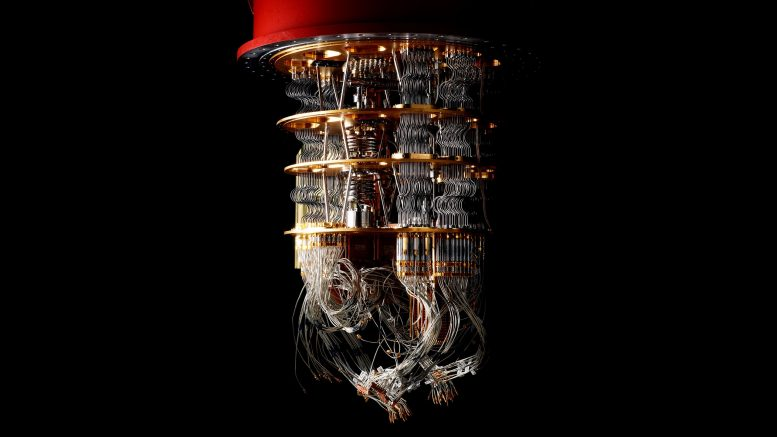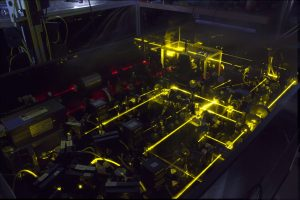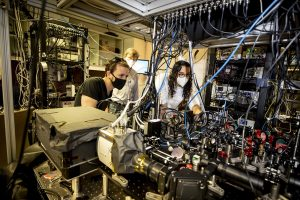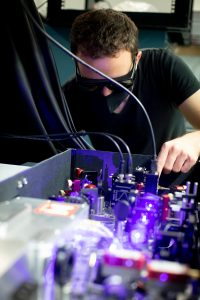# Quantum Startup Launches 256-qubit ComputerThe entanglement of qubits in a quantum computing system requires intense temperature control. Pictured is Google's cryostat holding the quanutm chip at nearly 0 Kelvin (absolute zero) | Source: Google

With numerous competitors across the technology industry, massive corporations such as Google and IBM have been at the forefront of developing larger and more complex systems of entangled qubits for their respective quantum computers. However, there are a few intrinsic drawbacks of quantum computation, both with constructing the systems as well as in writing the highly irregular algorithms to run on them. One quantum computing startup, QuEra, founded by physicists from Harvard, MIT, and Stanford, has sought to find a solution to these various problems with their new enhanced programable 256-qubit system.

Entanglement

The fundamental concept that allows for the exponentially beneficial processes of quantum computing is the physical state of entanglement that the qubit particles are trapped in. There are a variety of ways to entangle two particles, but the underlying principle is that in the observation of one of these qubits collapses the wave-function of not only one particle, but also the wave-function of the entangled qubit.Optical lattices are used across fields involving the trapping of quantum particles or atoms, requiring precise shifts or organization within the structure | Source: NIST

For example, a paired decay of one spin-$$0$$ particle that is stationary in the lab reference frame, into two leptons (spin-$$1/2$$ particles) yields two sets of entangled pieces of information. Because both traditional and angular momentum are conserved, the values of these two quantities are reliant on the other. Thus, if one has a momentum of $$15 \text{ Gev}/c$$ (in the lab reference frame) and a spin of $$1/2$$, then the other entangled particle must have a momentum of $$-15 \text{ Gev}/c$$ (in the lab reference frame) and a spin of $$-1/2$$. Therefore, the observation of one particle gives you the values of the other particle, exponentiating the efficiency of the processing time $$( 2^n$$ for $$n$$ entangled qubits with two possible states, or generally, $$c^n$$ for a system of $$n$$ entangled qubits with $$c$$ possible observable states, increasing the size of the Hilbert space$$)$$.

In this relationship, increasing the number of qubits drastically increases the number of potential pieces of information, allowing for immensely complex problems to be solved quickly through the direct observation of only a few qubits’ relevant quantities. As a result, the major firms of the quantum computing industry have been competing to increase the number of their entangled qubits within their scalable systems. However, sustaining entanglement becomes increasingly difficult as the size of the system increases, requiring immensely precise temperature and environment control. Otherwise, the information gathered during the computation will have interference from the unentangled qubits, yielding corrupted and useless data.

QuEra’s 256-qubit computer vastly outnumbers the previous record holders (Google’s 72-qubit chip “Bristlecone” and IBM’s 127-qubit chip “Eagle“) for entangled qubits in a quantum system, but it comes along with its own set of innate limitations and benefits.Scientists from Harvard and MIT collaborate through QuEra to build an industry leading 256-qubit quantum computer | Source: Harvard University

Programmability

Another major drawback that arises in quantum algorithm writing is the vast differences between certain classical algorithms and their quantum counterparts.

For example, when writing a discrete Fourier transform on a quantum system (dubbed the Quantum Fourier transform—QFT), the discrete transform is taken over the amplitudes of the wavefunctions. This technique is used in a variety of algorithmic situations such as Shor’s factoring algorithm as well as quantum phase estimation. The QFT transforms the computational (Pauli)-basis states into the Fourier-basis states. The single-qubit version of QFT is known as the Hadamard (or H) gate, which transforms between the orthogonal $$Z$$-basis $$(|0\rangle$$ and $$|1 \rangle)$$ to the $$X$$-basis states $$(|+\rangle$$ and $$|- \rangle)$$.

The intrinsic complications in the programmability of quantum computers arise in part from the physics, and thus, mathematics, of quantum mechanics, specifically the Dirac-notation linear algebra used to depict the quantum systems. As a result, only physicists well-versed in both physics and computer science techniques have the knowledge to develop properly functioning algorithms. Even then, the complicated nature of the expansive sets of gates and pathways derived from the exponentially organized systems yields a plethora of challenges in designing logical progressions and recursive elements.The use of $$420 \text{ mm}$$  optical tweezers allows for the precise placement of the atomic qubits in a variety of patterns, yielding a system that is easier to simulate and test | Source: Harvard

Optical Tweezers

Because the qubits of QuEra are laser-chilled atoms organized using an array of ultra-precise optical lattices (dubbed optical tweezers), they can be repositioned into different geometries that suit different algorithms better. Certain atoms retain enough of the properties of quantum mechanics that allow for the benefits of quantum computing, while still being large enough to be manipulated with these precise lasers. Other uses of optical tweezers include optical lattice (quantum) atomic clocks.

As a result, programming for these systems becomes more malleable, and allows for future scalability, meaning that when future quantum computers with more qubits are developed, the techniques used on the 256-qubit system remain relevant and useful. QuEra seeks to reach a 1000+ qubit computer by 2024, with the scalability of their system remaining relevant for years after.